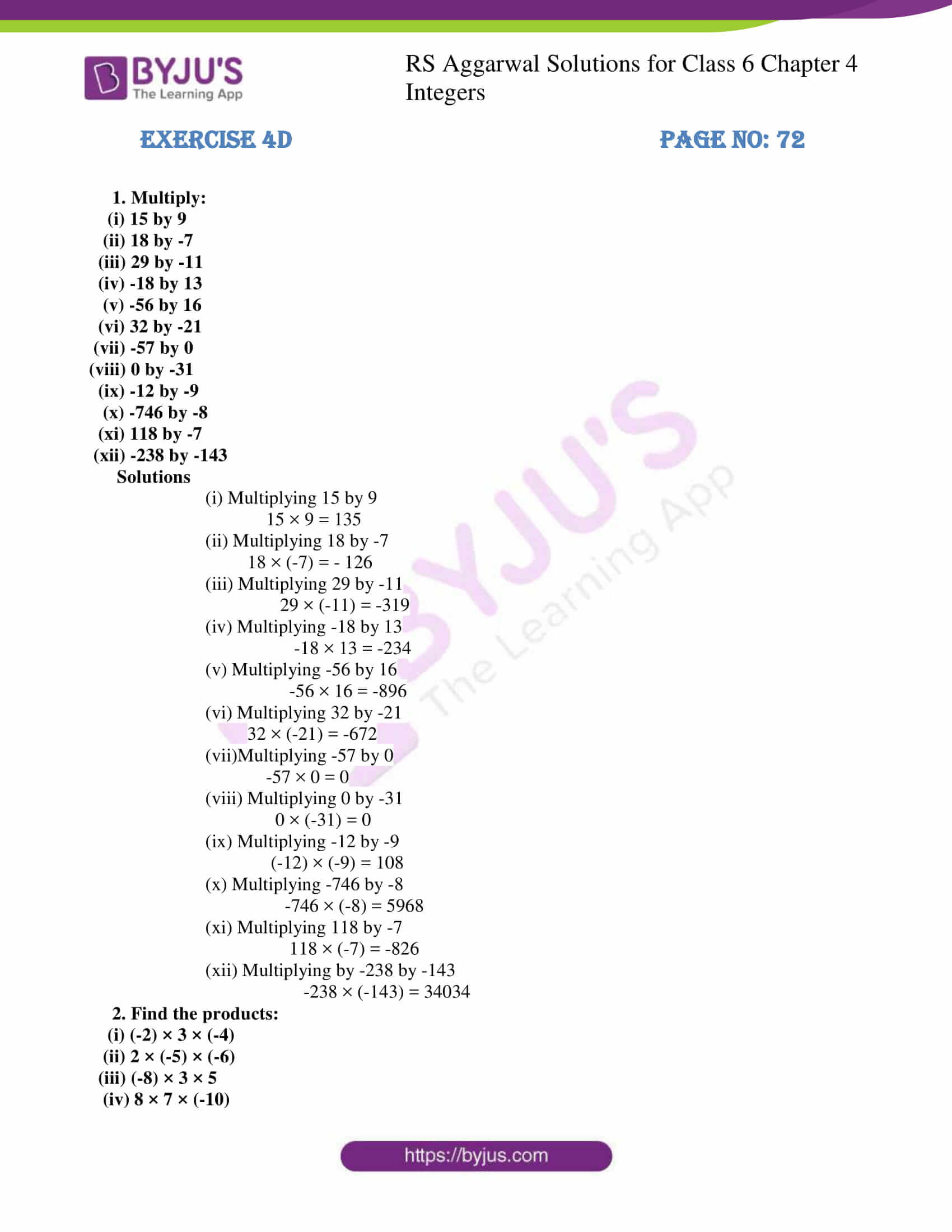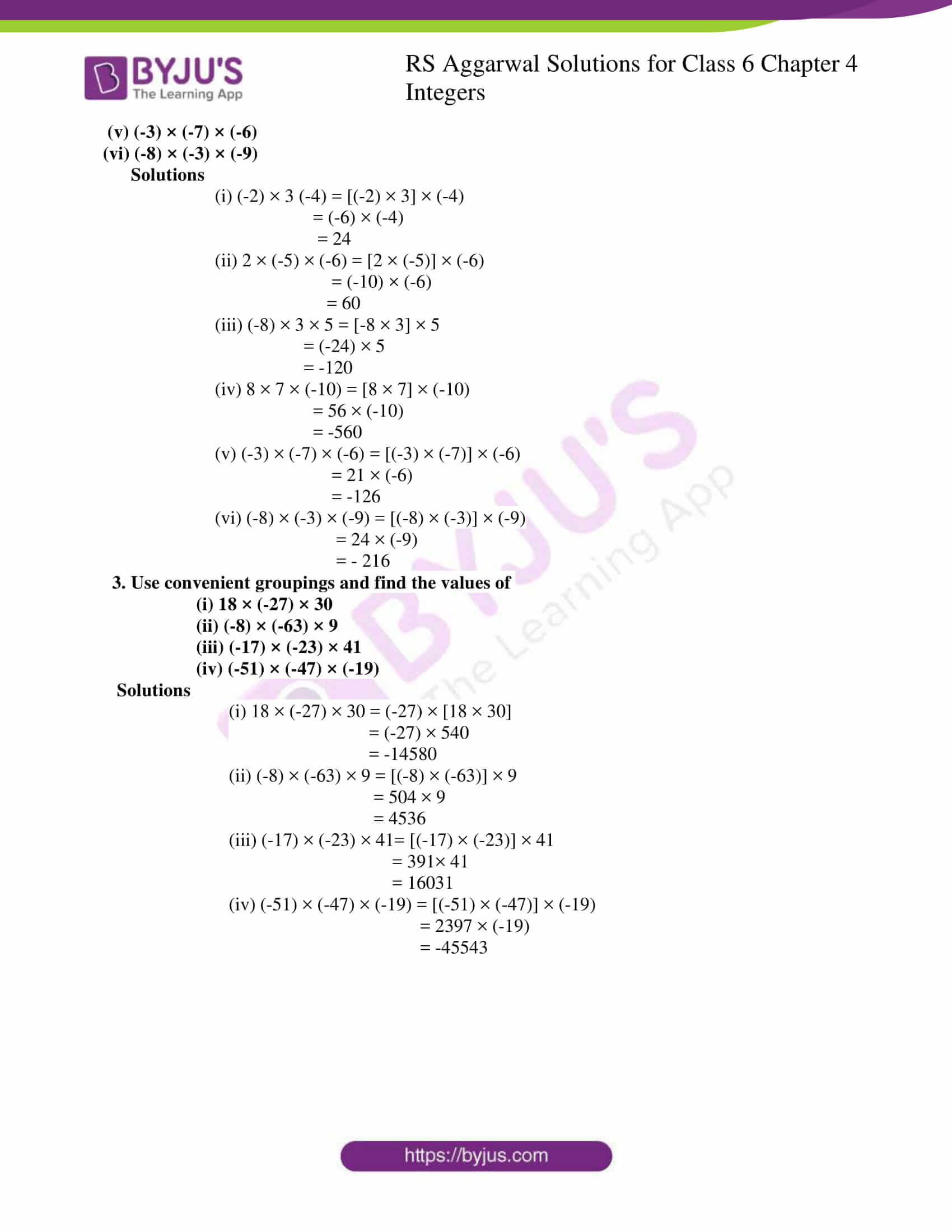# RS Aggarwal Solutions for Class 6 Chapter 4 Integers Exercise 4D

RS Aggarwal Solutions for Class 6 Chapter 4 Integers Exercise 4D are here in PDF format for the reference of the students. This exercise consists of topics like multiplication of Integers and properties of multiplication on integers. Students can practice questions based on the multiplication of Integers using RS Aggarwal Solutions as they give step by step solutions. Experts in Mathematics have prepared RS Aggarwal Solutions for class 6, which helps the students gain a better understanding of the concepts.

## Download PDF of RS Aggarwal Solutions for Class 6 Chapter 4 Integers Exercise 4D## Access answers to Maths RS Aggarwal Solutions for Class 6 Chapter 4 Integers Exercise 4D

1. Multiply:

(i) 15 by 9

(ii) 18 by -7

(iii) 29 by -11

(iv) -18 by 13

(v) -56 by 16

(vi) 32 by -21

(vii) -57 by 0

(viii) 0 by -31

(ix) -12 by -9

(x) -746 by -8

(xi) 118 by -7

(xii) -238 by -143

Solutions

(i) Multiplying 15 by 9

15 × 9 = 135

(ii) Multiplying 18 by -7

18 × (-7) = – 126

(iii) Multiplying 29 by -11

29 × (-11) = -319

(iv) Multiplying -18 by 13

-18 × 13 = -234

(v) Multiplying -56 by 16

-56 × 16 = -896

(vi) Multiplying 32 by -21

32 × (-21) = -672

(vii)Multiplying -57 by 0

-57 × 0 = 0

(viii) Multiplying 0 by -31

0 × (-31) = 0

(ix) Multiplying -12 by -9

(-12) × (-9) = 108

(x) Multiplying -746 by -8

-746 × (-8) = 5968

(xi) Multiplying 118 by -7

118 × (-7) = -826

(xii) Multiplying by -238 by -143

-238 × (-143) = 34034

2. Find the products:

(i) (-2) × 3 × (-4)

(ii) 2 × (-5) × (-6)

(iii) (-8) × 3 × 5

(iv) 8 × 7 × (-10)

(v) (-3) × (-7) × (-6)

(vi) (-8) × (-3) × (-9)

Solutions

(i) (-2) × 3 (-4) = [(-2) × 3] × (-4)

= (-6) × (-4)

= 24

(ii) 2 × (-5) × (-6) = [2 × (-5)] × (-6)

= (-10) × (-6)

= 60

(iii) (-8) × 3 × 5 = [-8 × 3] × 5

= (-24) × 5

= -120

(iv) 8 × 7 × (-10) = [8 × 7] × (-10)

= 56 × (-10)

= -560

(v) (-3) × (-7) × (-6) = [(-3) × (-7)] × (-6)

= 21 × (-6)

= -126

(vi) (-8) × (-3) × (-9) = [(-8) × (-3)] × (-9)

= 24 × (-9)

= – 216

3. Use convenient groupings and find the values of

(i) 18 × (-27) × 30

(ii) (-8) × (-63) × 9

(iii) (-17) × (-23) × 41

(iv) (-51) × (-47) × (-19)

Solutions

(i) 18 × (-27) × 30 = (-27) × [18 × 30]

= (-27) × 540

= -14580

(ii) (-8) × (-63) × 9 = [(-8) × (-63)] × 9

= 504 × 9

= 4536

(iii) (-17) × (-23) × 41= [(-17) × (-23)] × 41

= 391× 41

= 16031

(iv) (-51) × (-47) × (-19) = [(-51) × (-47)] × (-19)

= 2397 × (-19)

= -45543